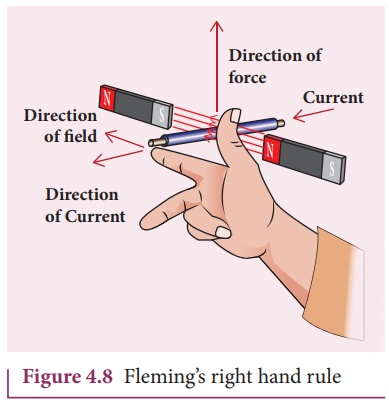Home | | Physics 12th Std | FlemingÔÇÖs right hand rule

# FlemingÔÇÖs right hand rule

FlemingÔÇÖs right hand rule is also known as generator rule.

FlemingÔÇÖs right hand rule

When a conductor moves in a magnetic field, the direction of motion of the conductor, the field and the induced current are given by FlemingÔÇÖs right hand rule and is as follows:The thumb, index finger and middle finger of right hand are stretched out in mutually perpendicular directions (as shown in Figure 4.8). If the index finger points the direction of the magnetic field and the thumb indicates the direction of motion of the conductor, then the middle finger will indicate the direction of the induced current.

FlemingÔÇÖs right hand rule is also known as generator rule.

EXAMPLE 4.6

If the current i flowing in the straight conducting wire as shown in the figure decreases, find out the direction of induced current in the metallic square loop placed near it.Solution

From right hand rule, the magnetic field by the straight wire is directed into the plane of the square loop perpendicularly and its magnetic flux is decreasing. The decrease in flux is opposed by the current induced in the loop by producing a magnetic field in the same direction as the magnetic field of the wire. Again from right hand rule, for this inward magnetic field, the direction of the induced current in the loop is clockwise.

EXAMPLE 4.7

The magnetic flux passes perpendicular to the plane of the circuit and is directed into the paper. If the magnetic flux varies with respect to time as per the following relation: ╬ŽB = (2t3 +3t2 + 8t +5) mWb , what is the magnitude of the induced emf in the loop when t = 3 s? Find out the direction of current through the circuit.Solution

╬ŽB = (2t 3 +3t2 + 8t +5) mWb; N =1; t = 3 s(ii) As time passes, the magnetic flux linked with the loop increases. According to LenzÔÇÖs law, the direction of the induced current should be in a way so as to oppose the flux increase. So, the induced current flows in such a way to produce a magnetic field opposite to the given field. This magnetic field is perpendicularly outwards. Therefore, the induced current flows in anti-clockwise direction.

Tags : Definition, Explanation, Solved Example Problems | Electromagnetic Induction , 12th Physics : Electromagnetic Induction and Alternating Current
Study Material, Lecturing Notes, Assignment, Reference, Wiki description explanation, brief detail
12th Physics : Electromagnetic Induction and Alternating Current : FlemingÔÇÖs right hand rule | Definition, Explanation, Solved Example Problems | Electromagnetic Induction